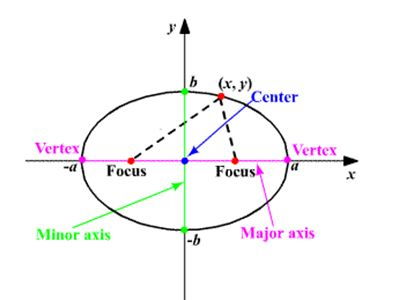# Conic Section: EllipseEllipseAn ellipse is the locus of the point such that its distance from the focus bears the constant ratio to the distance from the line called directrix .the value of eccentricity lies between the 0 and 1

Equation of an ellipse in standard form

$\frac{{\mathrm{x}}^{2}}{{\mathrm{a}}^{2}}\phantom{\rule{mediummathspace}{0ex}}+\frac{{\mathrm{y}}^{2}}{{\mathrm{b}}^{2}}\phantom{\rule{mediummathspace}{0ex}}$= 1

When the center of an ellipse is ( h, k)

$\frac{{\left(\mathrm{x}-\mathrm{h}\right)}^{2}}{{\mathrm{a}}^{2}}\phantom{\rule{mediummathspace}{0ex}}+\frac{{\left(\mathrm{y}-\mathrm{k}\right)}^{2}}{{\mathrm{b}}^{2}}\phantom{\rule{mediummathspace}{0ex}}$= 1

Hyperbola

A hyperbola is a set of all points in a plane such that the difference of the distances from the two fixed points called foci is a constant.Equation of the hyperbola in a standard form is given by$\frac{{\mathrm{x}}^{2}}{{\mathrm{a}}^{2}}\phantom{\rule{mediummathspace}{0ex}}-\phantom{\rule{mediummathspace}{0ex}}\frac{{\mathrm{y}}^{2}}{{\mathrm{b}}^{2}}\phantom{\rule{mediummathspace}{0ex}}$= 1 centre at origin C (0, 0) foci = (± ae, 0) directrix ,x =± $\frac{\mathrm{a}}{\mathrm{e}}$

Length of latus rectum =